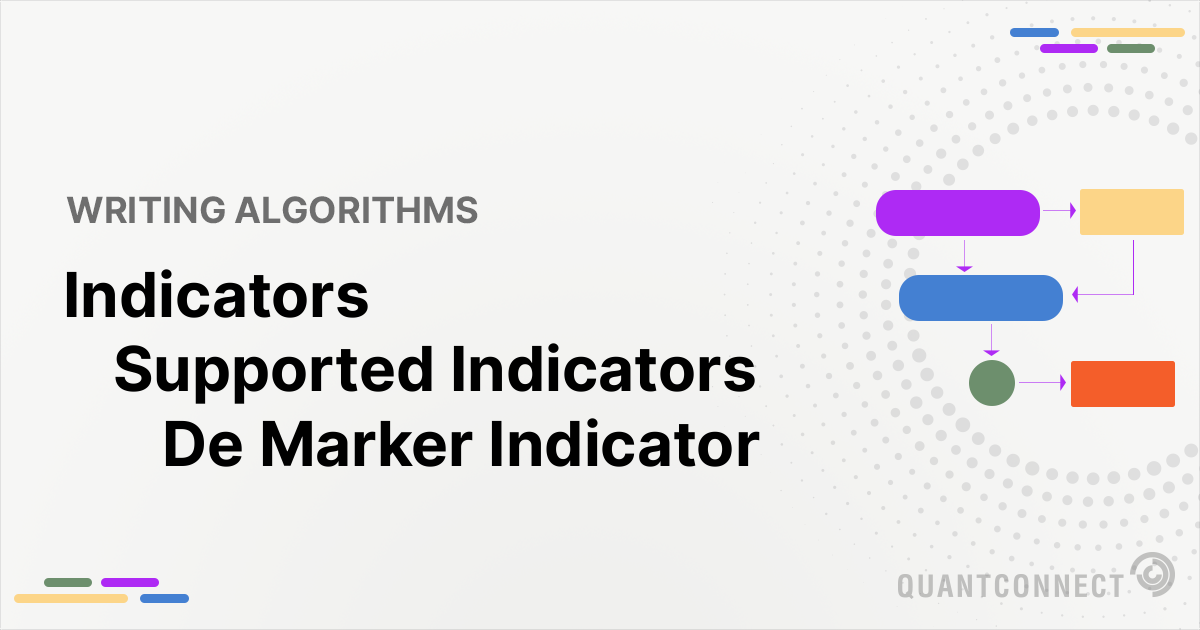# Supported Indicators

## De Marker Indicator

### Introduction

In the DeMarker strategy, for some period of size N, set: DeMax = High - Previous High, and DeMin = Previous Low - Low where, in the prior, if either term is less than zero (DeMax or DeMin), set it to zero. We can now define the indicator itself, DEM, as: DEM = MA(DeMax)/(MA(DeMax)+MA(DeMin)) where MA denotes a Moving Average of period N. https://www.investopedia.com/terms/d/demarkerindicator.asp

To view the implementation of this indicator, see the LEAN GitHub repository.

### Using DEM Indicator

To create an automatic indicators for DeMarkerIndicator, call the DEM helper method from the QCAlgorithm class. The DEM method creates a DeMarkerIndicator object, hooks it up for automatic updates, and returns it so you can used it in your algorithm. In most cases, you should call the helper method in the Initialize method.

public class DeMarkerIndicatorAlgorithm : QCAlgorithm
{
private Symbol _symbol;
private DeMarkerIndicator _dem;

public override void Initialize()
{
_dem = DEM(_symbol, 20, MovingAverageType.Simple);
}

public override void OnData(Slice data)
{
{
// The current value of _dem is represented by itself (_dem)
// or _dem.Current.Value
Plot("DeMarkerIndicator", "dem", _dem);

}
}
}
class DeMarkerIndicatorAlgorithm(QCAlgorithm):
def Initialize(self) -> None:
self.dem = self.DEM(self.symbol, 20, MovingAverageType.Simple)

def OnData(self, slice: Slice) -> None:
# The current value of self.dem is represented by self.dem.Current.Value
self.Plot("DeMarkerIndicator", "dem", self.dem.Current.Value)



The following reference table describes the DEM method:

### DEM()1/1

            DeMarkerIndicator QuantConnect.Algorithm.QCAlgorithm.DEM (
Symbol                            symbol,
Int32                             period,
MovingAverageType                 type,
*Nullable<Resolution>       resolution,
*Func<IBaseData, TradeBar>  selector
)


Creates a new DeMarker Indicator (DEM), an oscillator-type indicator measuring changes in terms of an asset's High and Low tradebar values.

If you don't provide a resolution, it defaults to the security resolution. If you provide a resolution, it must be greater than or equal to the resolution of the security. For instance, if you subscribe to hourly data for a security, you should update its indicator with data that spans 1 hour or longer.

The following table describes the MovingAverageType enumeration members:

You can manually create a DeMarkerIndicator indicator, so it doesn't automatically update. Manual indicators let you update their values with any data you choose.

Updating your indicator manually enables you to control when the indicator is updated and what data you use to update it. To manually update the indicator, call the Update method with a TradeBar, or QuoteBar. The indicator will only be ready after you prime it with enough data.

public class DeMarkerIndicatorAlgorithm : QCAlgorithm
{
private Symbol _symbol;
private DeMarkerIndicator _dem;

public override void Initialize()
{
_dem = new DeMarkerIndicator(20, MovingAverageType.Simple);
}

public override void OnData(Slice data)
{
if (data.Bars.TryGeValue(_symbol, out var bar))
{
_dem.Update(bar);
}

{
// The current value of _dem is represented by itself (_dem)
// or _dem.Current.Value
Plot("DeMarkerIndicator", "dem", _dem);

}
}
}
class DeMarkerIndicatorAlgorithm(QCAlgorithm):
def Initialize(self) -> None:
self.dem = DeMarkerIndicator(20, MovingAverageType.Simple)

def OnData(self, slice: Slice) -> None:
bar = slice.Bars.get(self.symbol)
if bar:
self.dem.Update(bar)

# The current value of self.dem is represented by self.dem.Current.Value
self.Plot("DeMarkerIndicator", "dem", self.dem.Current.Value)



To register a manual indicator for automatic updates with the security data, call the RegisterIndicator method.

public class DeMarkerIndicatorAlgorithm : QCAlgorithm
{
private Symbol _symbol;
private DeMarkerIndicator _dem;

public override void Initialize()
{
_dem = new DeMarkerIndicator(20, MovingAverageType.Simple);
RegisterIndicator(_symbol, _dem, Resolution.Daily);
}

public override void OnData(Slice data)
{
{
// The current value of _dem is represented by itself (_dem)
// or _dem.Current.Value
Plot("DeMarkerIndicator", "dem", _dem);

}
}
}
class DeMarkerIndicatorAlgorithm(QCAlgorithm):
def Initialize(self) -> None:
self.dem = DeMarkerIndicator(20, MovingAverageType.Simple)
self.RegisterIndicator(self.symbol, self.dem, Resolution.Daily)

def OnData(self, slice: Slice) -> None:
# The current value of self.dem is represented by self.dem.Current.Value
self.Plot("DeMarkerIndicator", "dem", self.dem.Current.Value)



The following reference table describes the DeMarkerIndicator constructor:

### DeMarkerIndicator()1/2

            DeMarkerIndicator QuantConnect.Indicators.DeMarkerIndicator (
int                 period,
*MovingAverageType  type
)


Initializes a new instance of the DeMarkerIndicator class with the specified period.

### DeMarkerIndicator()2/2

            DeMarkerIndicator QuantConnect.Indicators.DeMarkerIndicator (
string              name,
int                 period,
*MovingAverageType  type
)


Initializes a new instance of the DeMarkerIndicator class with the specified name and period.

### Visualization

The following image shows plot values of selected properties of DeMarkerIndicator using the plotly library.You can also see our Videos. You can also get in touch with us via Discord.